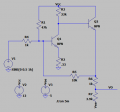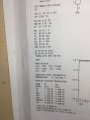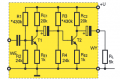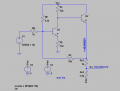# Transistor circuit

#### ericgibbs

Joined Jan 29, 2010
9,114
hi Z,
As you use LTSpice, why not create the circuit in the simulator.?
E

•Zeeus

#### Jony130

Joined Feb 17, 2009
5,090
•Zeeus

#### Zeeus

Joined Apr 17, 2019
481
hi Z,
As you use LTSpice, why not create the circuit in the simulator.?
E
Yes will use spice : just wanted to understand the theory.. The book uses spice to get Dc values
The easiest way to find the voltage gain is to use a circuit simulator. Buy because Re1 = 330kΩ and RL = 22kΩ.
This circuit will do not have any voltage gain. It is more like an attenuator.

And what you exactly do not understand?

Also, see the example here
https://electronics.stackexchange.com/questions/372891/calculating-the-transresistance-in-a-multistage-voltage-shuntshunt-shunt-feedb/372948#372948

And the name of a book is?
Hi : okay thanks... You can do not easiest way?
Thanks : the link similar problem

book : handbook of analog circuit design : Feucht
You have it

#### Attachments

• 2.8 KB Views: 11
Last edited:

#### Jony130

Joined Feb 17, 2009
5,090
OK. so what you want to know?

Also, I'm 100% sure that there's an error in RE value in a book, It cannot be 330kΩ. So please check this.

•Zeeus

#### Zeeus

Joined Apr 17, 2019
481
OK. so what you want to know?

Also, I'm 100% sure that there's an error in RE value in a book, It cannot be 330kΩ. So please check this.

#### ronsimpson

Joined Oct 7, 2019
344
Buy because Re1 = 330kΩ and RL = 22kΩ.
I think there is an error(s) in the book.
First: No gain?? I think 330k should be 330 ohms. Now we have gain.
Second: Bias is not right, never was. 6.1k should be 61k
In SPICE I changes the 1V signal on input to 0.5V.
Now the circuit is just strange.

Now Ve2 is at 6.8V or about 1/2 of the 12V supply. That is good.
I think "Vo" is the output not Ve2. Also strange.
With a 0.5V signal in Vin we get Ve2=about 4V and VO=3V peak.

If I was making this amplifier: (note 0.33 ohms could be 0)•Zeeus

#### Audioguru again

Joined Oct 21, 2019
542
The same current is in R2 as in R3. Since R2 has a value that is much higher than R3 then the signal at R3 is much lower than the signal at R2.

#### Zeeus

Joined Apr 17, 2019
481
wow thanks people..Almost thought was on "ignore list"

@ronsimpson 1 like for you : but did you notice there is distortion with your changes?

How did you get dc value? wait though : want to read again and try...will ask later

Last edited:

#### Jony130

Joined Feb 17, 2009
5,090
Last edited:
•Zeeus

#### Zeeus

Joined Apr 17, 2019
481
No, I don't have this book. But from what I see on your photo on the left sit there is a spice netlist.
Can you show it?

And here you have some examples:

But I assume that you know how to calculate the gain of a multistage amplifier?
lol..You recommend book you do not have : anyways, nice book : thanks..just 3.9 hard to follow (hard is good?)#### Jony130

Joined Feb 17, 2009
5,090
lol..You recommend book you do not have
When and where I recommend buying this book?

Quick test:

The voltage gain if this amplifier is?As for your circuit from the netlist, I see that RE has a value of 330Ω and that the Vcc value is not equal to +12V by Vcc = +5V instead.
also, the BJT current gain is β = 99. Therefore the correct circuit should look like this:First, notice that this circuit can be treated as an opamp based inverting amplifier with the gain RF/RB but because we have only one CE stage that provides a voltage gain (RC/RE ≈ 66 V/V) the overall voltage gain will be less than 10V/V. Also, the voltage divider at Q2 emitter will reduce the gain further (3.9/4.9 ≈ 0.79). So, the ballpark figure of a gain is Av ≈ 66/(1 + 66*1/10)*0.79 ≈ 6.8 V/V

#### Attachments

• 1.7 KB Views: 3
• 62.6 KB Views: 3
Last edited:
•Zeeus

#### Zeeus

Joined Apr 17, 2019
481
Quick test:

The voltage gain if this amplifier is?
View attachment 194082
lol thanks...doing..less 10mins maybe
will assume U is 12

yh?

"First, notice that this circuit can be treated as an opamp based inverting amplifier" Yes this guideline for me

Last edited:

#### Jony130

Joined Feb 17, 2009
5,090
lol thanks...doing..less 10mins maybe
will assume U is 12

Forgot about Vcc, just simply assume β = 100 and ignore re << RE1 and RE2.
The first stage gain is?

#### Zeeus

Joined Apr 17, 2019
481
Forgot about Vcc, just simply assume β = 100 and ignore re << RE1 and RE2.
The first stage gain is?
16.2? it's not 20

#### Jony130

Joined Feb 17, 2009
5,090

#### Zeeus

Joined Apr 17, 2019
481
Why 16.2? Can you show me the calculations?
hmmm...why not? okay

oh and -16.2 meant to say

Think this wrong...

Rb is 24k||430k

$a = β/ (β+1)$

Vi : input voltage Ie = emitter current

$Ie (Re + Rb/ (β + 1)) = Vi$

$Vo = -a*Ie * 20k$

from this then found $Vo/Vi$
Mistake?

Last edited:

#### Zeeus

Joined Apr 17, 2019
481
Latex works
View attachment 194091

$I_L = \frac{V_1}{R_1}$
Is the gain correct? if wrong : think can do another calculation$x/y + c = 4$

"When and where I recommend buying this book?"
To answer this : Bordodynov posted a circuit with diff amp input : there was a capacitor placed between both emitters
DickCappels enquired about the circuit and you posted the book with the circuit. Assumed you had the book so got it (you can give me refund? : joke)

Last edited: